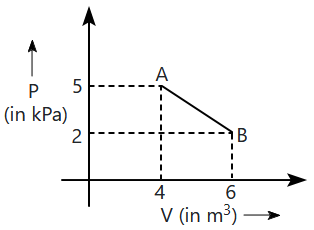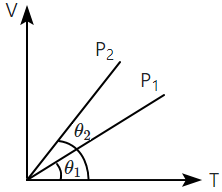Two vessels separately contain two ideal gases A and B at the same temperature, the pressure of A being twice that of B. Under such conditions, the density of A is found to be 1.5 times the density of B. The ratio of molecular weight of A and B is:

1. $\frac{2}{3}$

2. $\frac{3}{4}$

3. 2

4. $\frac{1}{2}$

Subtopic:  Ideal Gas Equation |
To view explanation, please take trial in the course below.
NEET 2023 - Target Batch - Aryan Raj Singh
To view explanation, please take trial in the course below.
NEET 2023 - Target Batch - Aryan Raj Singh
Please attempt this question first.
Launched MCQ Practice Books

Prefer Books for Question Practice? Get NEETprep's Unique MCQ Books with Online Video/Text Solutions via Telegram Bot

One mole of an ideal diatomic gas undergoes a transition from A to B along a path AB as shown in the figure.The change in internal energy of the gas during the transition is:

1. 20 kJ
2. - 20 kJ
3. 20 J
4. -12 kJ

Subtopic:  Law of Equipartition of Energy |
To view explanation, please take trial in the course below.
NEET 2023 - Target Batch - Aryan Raj Singh
To view explanation, please take trial in the course below.
NEET 2023 - Target Batch - Aryan Raj Singh
Please attempt this question first.
Launched MCQ Practice Books

Prefer Books for Question Practice? Get NEETprep's Unique MCQ Books with Online Video/Text Solutions via Telegram Bot

The ratio of the specific heats $\frac{{\mathrm{C}}_{\mathrm{p}}}{{\mathrm{C}}_{\mathrm{v}}}=\mathrm{\gamma }$ in terms of degrees of freedom(n) is given by:

1. $\left(1+\frac{1}{\mathrm{n}}\right)$
2. $\left(1+\frac{\mathrm{n}}{3}\right)$
3. $\left(1+\frac{2}{\mathrm{n}}\right)$
4. $\left(1+\frac{\mathrm{n}}{2}\right)$

Subtopic:  Specific Heat |
To view explanation, please take trial in the course below.
NEET 2023 - Target Batch - Aryan Raj Singh
To view explanation, please take trial in the course below.
NEET 2023 - Target Batch - Aryan Raj Singh
Please attempt this question first.
Launched MCQ Practice Books

Prefer Books for Question Practice? Get NEETprep's Unique MCQ Books with Online Video/Text Solutions via Telegram Bot

The mean free path of molecules of a gas (radius 'r') is inversely proportional to:

1. ${r}^{3}$

2. ${r}^{2}$

3. r

4. $\sqrt{r}$

Subtopic:  Mean Free Path |
To view explanation, please take trial in the course below.
NEET 2023 - Target Batch - Aryan Raj Singh
To view explanation, please take trial in the course below.
NEET 2023 - Target Batch - Aryan Raj Singh
Please attempt this question first.
Launched MCQ Practice Books

Prefer Books for Question Practice? Get NEETprep's Unique MCQ Books with Online Video/Text Solutions via Telegram Bot

In the given (V–T) diagram, what is the relation between pressure P1 and P21. P> P1
2. P< P1
3. Cannot be predicted
4. P= P1

Subtopic:  Ideal Gas Equation |
To view explanation, please take trial in the course below.
NEET 2023 - Target Batch - Aryan Raj Singh
To view explanation, please take trial in the course below.
NEET 2023 - Target Batch - Aryan Raj Singh
Please attempt this question first.
Launched MCQ Practice Books

Prefer Books for Question Practice? Get NEETprep's Unique MCQ Books with Online Video/Text Solutions via Telegram Bot

The amount of heat energy required to raise the temperature of 1 g of Helium at NTP, from TK to TK is:

1. $\frac{3}{2}{\mathrm{N}}_{\mathrm{a}}{\mathrm{k}}_{\mathrm{B}}\left({\mathrm{T}}_{2}-{\mathrm{T}}_{1}\right)$

2. $\frac{3}{4}{\mathrm{N}}_{\mathrm{a}}{\mathrm{k}}_{\mathrm{B}}\left({\mathrm{T}}_{2}-{\mathrm{T}}_{1}\right)$

3. $\frac{3}{4}{\mathrm{N}}_{\mathrm{a}}{\mathrm{k}}_{\mathrm{B}}\frac{{\mathrm{T}}_{2}}{{\mathrm{T}}_{1}}$

4. $\frac{3}{8}{\mathrm{N}}_{\mathrm{a}}{\mathrm{k}}_{\mathrm{B}}\left({\mathrm{T}}_{2}-{\mathrm{T}}_{1}\right)$

Subtopic:  Specific Heat |
To view explanation, please take trial in the course below.
NEET 2023 - Target Batch - Aryan Raj Singh
To view explanation, please take trial in the course below.
NEET 2023 - Target Batch - Aryan Raj Singh
Please attempt this question first.
Launched MCQ Practice Books

Prefer Books for Question Practice? Get NEETprep's Unique MCQ Books with Online Video/Text Solutions via Telegram Bot

If Cp and Cv denote the specific heats (per unit mass) of an ideal gas of molecular weight M (where R is the molar gas constant), the correct relation is

1. Cp – Cv = R

2. Cp – Cv = R / M

3. Cp – Cv = MR

4. Cp – Cv = R / M2

Subtopic:  Specific Heat |
To view explanation, please take trial in the course below.
NEET 2023 - Target Batch - Aryan Raj Singh
To view explanation, please take trial in the course below.
NEET 2023 - Target Batch - Aryan Raj Singh
Please attempt this question first.
Launched MCQ Practice Books

Prefer Books for Question Practice? Get NEETprep's Unique MCQ Books with Online Video/Text Solutions via Telegram Bot

At 10C the value of the density of a fixed mass of an ideal gas divided by its pressure is x. At 110oC this ratio is:

1.  $\mathrm{x}$

2.  $\frac{383}{283}\mathrm{x}$

3.  $\frac{10}{110}\mathrm{x}$

4.  $\frac{283}{383}\mathrm{x}$

Subtopic:  Ideal Gas Equation |
To view explanation, please take trial in the course below.
NEET 2023 - Target Batch - Aryan Raj Singh
To view explanation, please take trial in the course below.
NEET 2023 - Target Batch - Aryan Raj Singh
Please attempt this question first.
Launched MCQ Practice Books

Prefer Books for Question Practice? Get NEETprep's Unique MCQ Books with Online Video/Text Solutions via Telegram Bot